# Buffon problem

(diff) ← Older revision | Latest revision (diff) | Newer revision → (diff)

of the needle

A classical problem in the theory of geometric probabilities, which is rightly considered to be the starting point in the development of this theory. First posed by G. Buffon in 1733 and reproduced together with its solution in . Buffon considered the following situation: A needle of length, where, is thrown at random on a plane ruled by parallel lines at a distance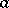from one another. What is the probability that the needle thus thrown will come to rest across one of the lines? Clearly, the location of the needle is defined by the distancefrom its centre to the nearest straight line and by the acute anglebetween the needle and the perpendicular to the line. The magnitudelies between zero and, whilelies between zero and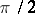. It is assumed that the point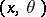is uniformly distributed in the appropriate rectangle (this is equivalent to assuming that the random variablesandare independent and are uniformly distributed overand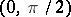). Then the sought probability is defined as the ratio between the areas corresponding to the favourable and to all possible results, and is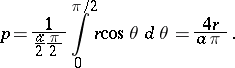(*)

In the past Buffon's problem served as the tool for the experimental check on the Bernoulli theorem. In fact, if the needle is throwntimes, and crosses one of the lines incases, the frequencyshould, for large values of, according to Bernoulli's theorem, be close to the probability (*). This idea was used by many workers for the determination of the numberby random trials , . Buffon also considered other similar problems, in particular the problem of the needle crossing the lines belonging to two mutually-perpendicular systems which subdivide the plane into rectangles having sides of lengthsandrespectively. The answer given by Buffon to this problem is incorrect. The correct solutionwas found by P. Laplace in 1812.

How to Cite This Entry:
Buffon problem. Encyclopedia of Mathematics. URL: http://encyclopediaofmath.org/index.php?title=Buffon_problem&oldid=12713
This article was adapted from an original article by A.V. Prokhorov (originator), which appeared in Encyclopedia of Mathematics - ISBN 1402006098. See original article